# 深度学习100问之深入理解Regularization（正则化）

## 声明

1）该文章整理自网上的大牛和机器学习专家无私奉献的资料，具体引用的资料请看参考文献。
2）本文仅供学术交流，非商用。所以每一部分具体的参考资料并没有详细对应。如果某部分不小心侵犯了大家的利益，还望海涵，并联系博主删除。
3）博主才疏学浅，文中如有不当之处，请各位指出，共同进步，谢谢。
4）此属于第一版本，若有错误，还需继续修正与增删。还望大家多多指点。大家都共享一点点，一起为祖国科研的推进添砖加瓦。

## 1、什么是正则化？• 红色这条想象力过于丰富且上下横跳的曲线就是 过拟合
• 绿色这条相对平滑的曲线就是正常拟合。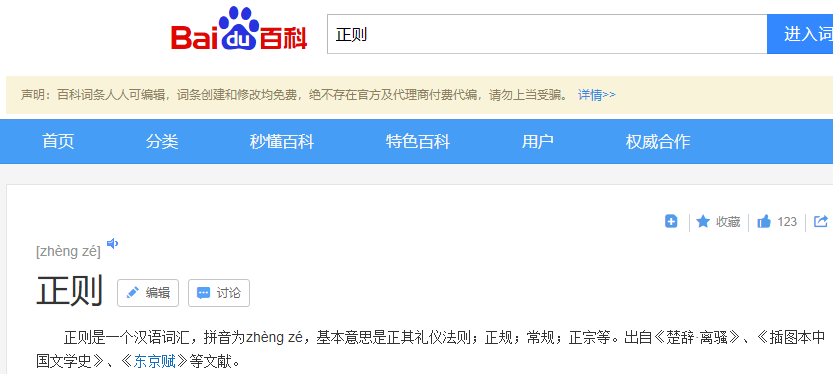## 2、如何正则化？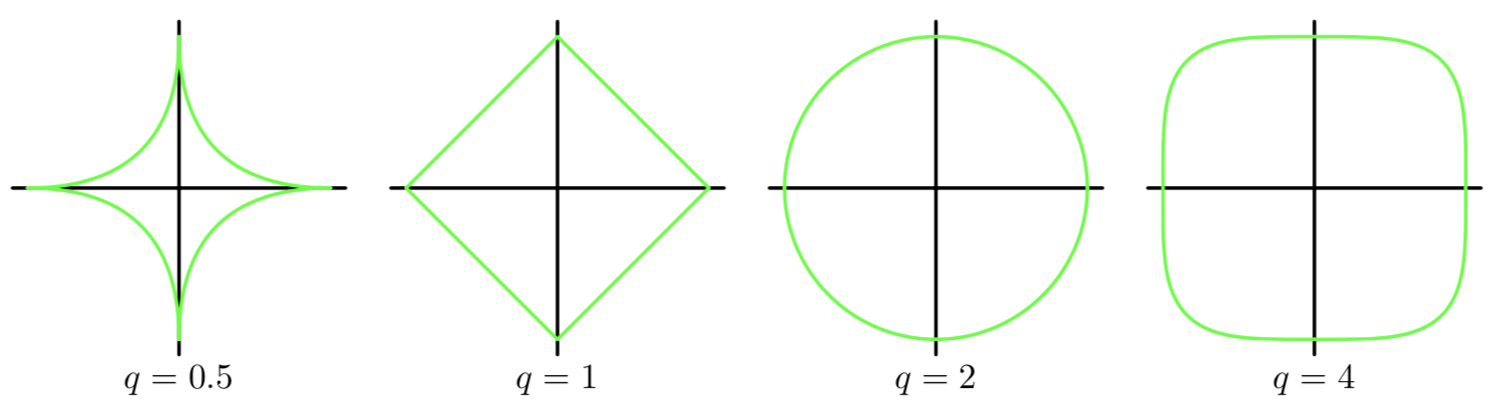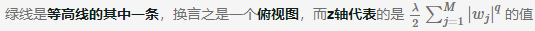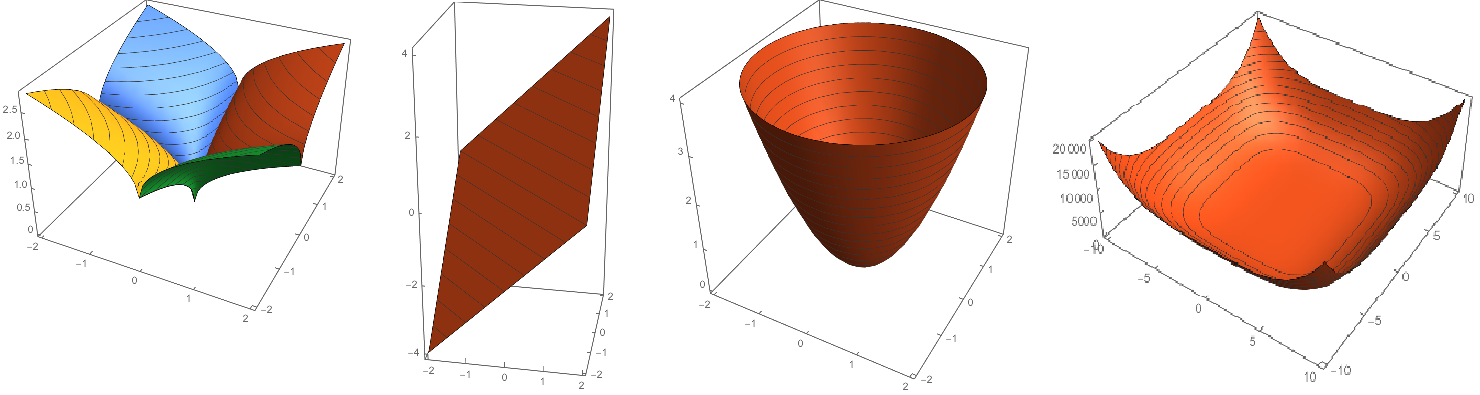## 3、什么是范数？### 1）∞ - 范数### 2）1 - 范数### 3）2 - 范数## 4、L1 正则化和 L2 正则化

### 1）L1 正则化

L1 参数范数惩罚通常称为 LassoL1 正则化策略通过在目标函数中添加一个正则化项来使权重更接近原点。
$\Omega(\boldsymbol{\theta})=\frac{1}{2}\|\boldsymbol{w}\|_{1}$### 2）L2 正则化

L2 参数范数惩罚通常称为 岭回归L2 策略通过在目标函数中添加一个正则化项，使权重更接近原点。

$\Omega(\boldsymbol{\theta})=\frac{1}{2}\|\boldsymbol{w}\|_{2}^{2}$### 3）小结

L1 和 L2 的下降速度如下：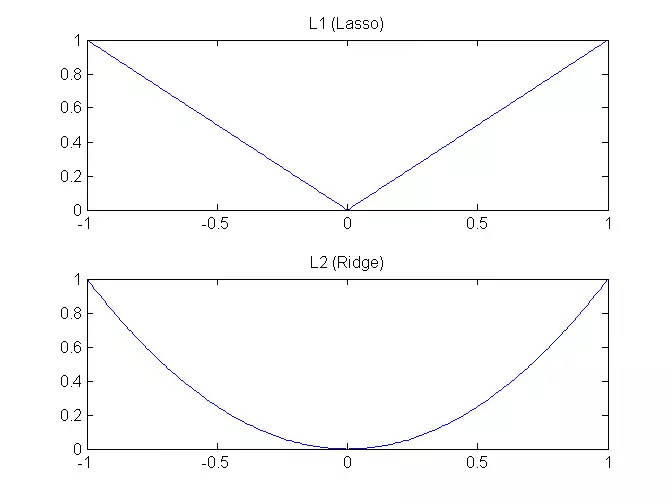L1 和 L2 的模型空间如下：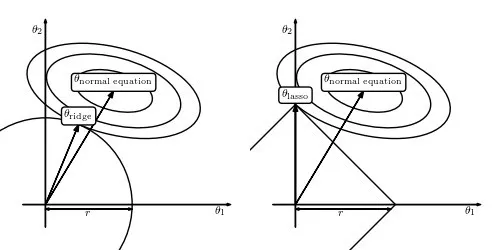## 5、神经网络的正则化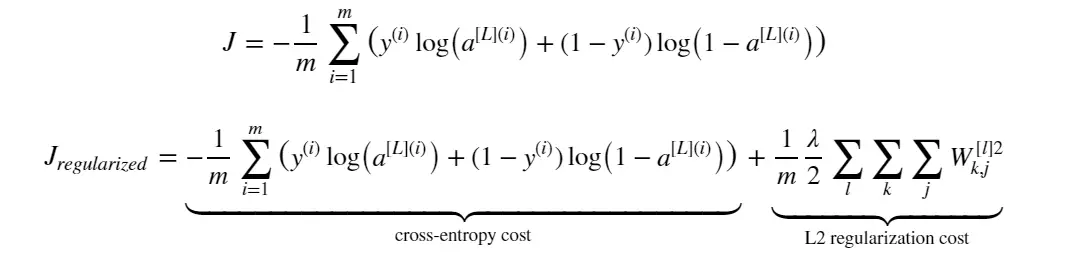# 用 L2 正则化（L2 regularization）实现代价函数
def compute_cost_with_regularization(A3, Y, parameters, lambd):
"""
Arguments:
A3 -- 激活函数后，前向传播的输出，形状（output size, number of examples）
Y -- "true"标签向量，形状（output size, number of examples）
parameters -- 包含模型参数的python字典（dictionary）
lambd -- 正则化超参数，标量

Returns:
cost - 正则化损失函数的损失函数值
"""
m = Y.shape
W1 = parameters["W1"]
W2 = parameters["W2"]
W3 = parameters["W3"]

# 这就得到了损失函数的交叉熵部分
cross_entropy_cost = compute_cost(A3, Y)

L2_regularization_cost = 1 / m * lambd / 2 * \
(np.sum(np.square(W1)) + np.sum(np.square(W2)) + np.sum(np.square(W3)))

cost = cross_entropy_cost + L2_regularization_cost

return cost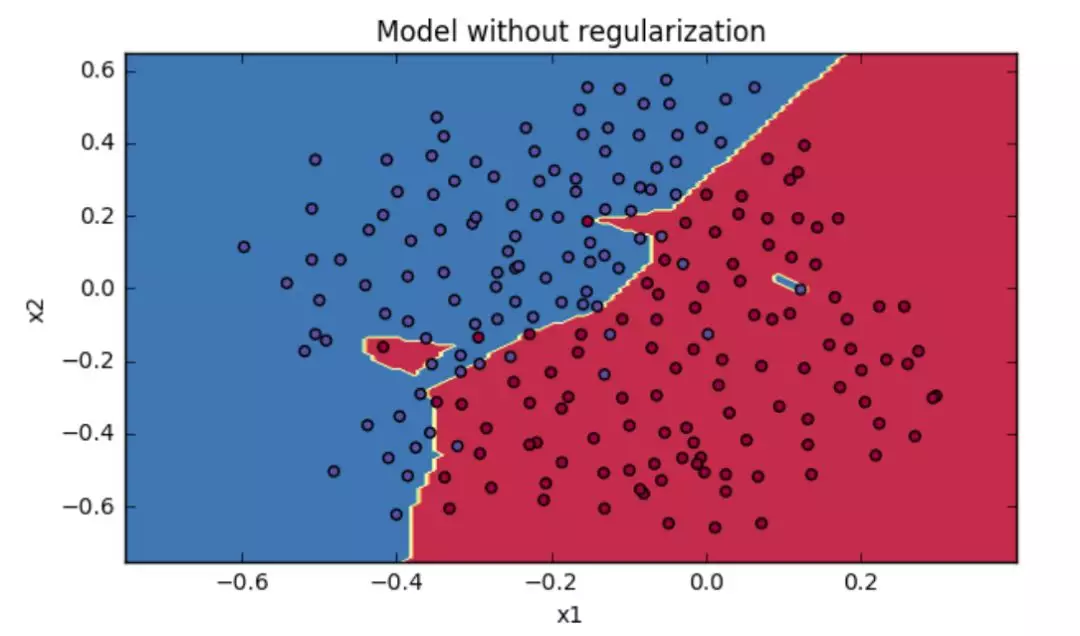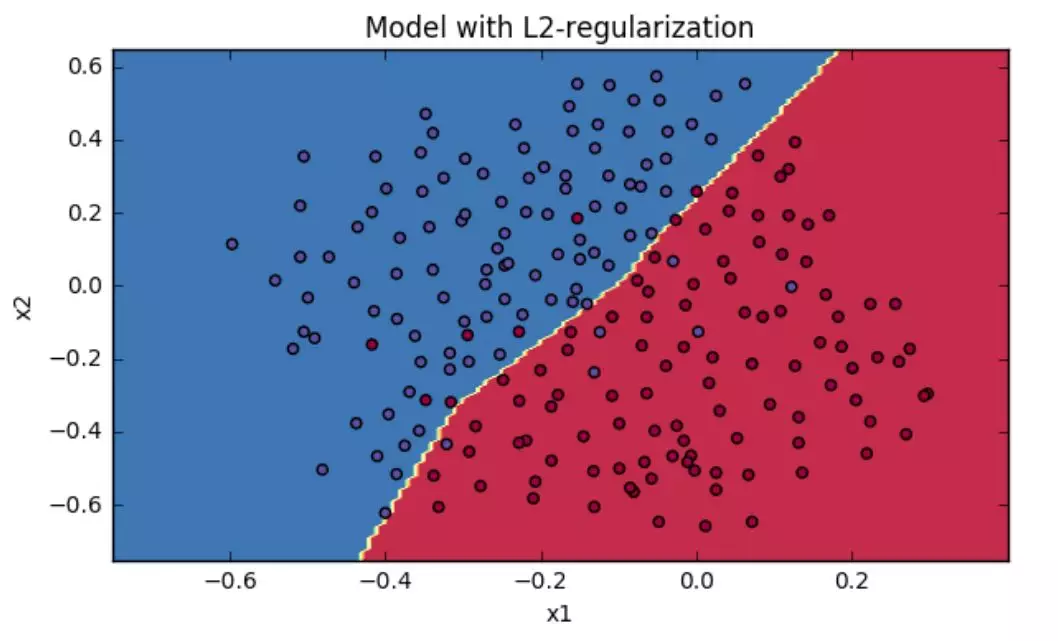## 参考文章